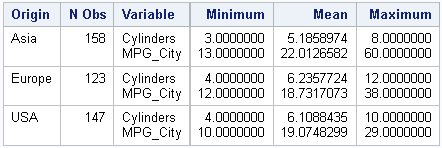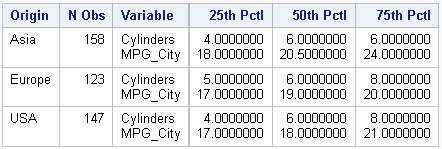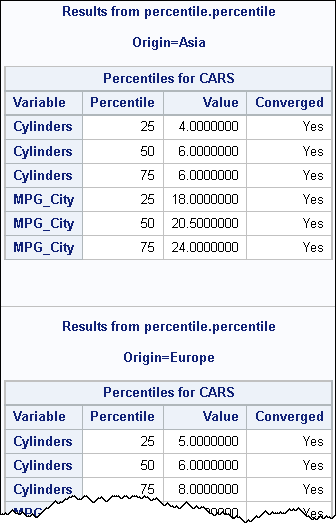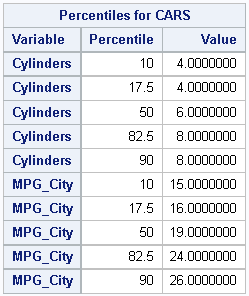How can you estimate percentiles in SAS Viya? This article shows how to call the percentile action from PROC CAS to estimate percentiles of variables in a CAS data table. Percentiles and quantiles are essentially the same (the pth quantile is the 100*pth percentile for p in [0, 1]), so this article also shows how to estimate quantiles in SAS Viya.

I previously mentioned that some SAS procedures are CAS-enabled, which means that they will call a CAS action that runs on the CAS server if you specify a CAS table on the DATA= option. However, I also mentioned that some Base SAS procedures are "hybrid," which means that they might run on the CAS server or on the SAS Compute Server. But be careful: some Base SAS procedures (including the DATA step) look at the options that you specify to decide whether they can process the data on the CAS server. If not, they will pull the data down from the server to the SAS client and compute the result there. This is probably not what you want. It is inefficient to copy data from the CAS server if you can perform the computation on the server where the data are.

### PROC MEANS is a hybrid procedure

A good example of a hybrid procedure is PROC MEANS. If the data are in a CAS table, PROC MEANS will look at the options that you specify. If you request basic descriptive statistics (N, MIN, MAX, MEAN, STD, etc), the procedure will call the aggregate action and compute the statistics in CAS. However, for some procedure options (and, I confess, I don't know which ones) the procedure decides that the requested statistics should be computed on the SAS client and uses the CAS LIBNAME engine to access the data. This results in reading the data from the CAS table and performing the computation on the SAS client.

Let's construct an example for which PROC MEANS computes the statistics in CAS. The following program loads the data in the Sashelp.Cars data set into a CAS table. It then calls PROC MEANS to compute descriptive statistics:

```cas; /* connect to CAS server */ libname mylib cas; /* active caslib, whatever it is */   proc casutil; load data=Sashelp.Cars casout='Cars' replace; /* load data into the active caslib */ quit;   proc means data=mylib.Cars nolabels min mean max; /* runs action on CAS server */ class Origin; vars Cylinders MPG_City; run;```The procedure outputs a familiar table that displays the mean, min, and max statistics for two variables, each grouped according to levels of the classification variable. In addition, the procedure displays the following note in the log:

`NOTE: The CAS aggregation.aggregate action will be used to perform the initial summarization.`

This note informs you that the computation took place on the CAS server. It also tells you that PROC MEANS used the aggregate action to perform the computation.

The behavior of PROC MEANS changes if you request percentiles. For some reason (unknown to me), the percentiles are not computed on the CAS server. Instead, the data are read from the CAS table by using the CAS libname engine, and the computation takes place on the SAS client:

```proc means data=mylib.Cars nolabels P25 P50 P75; /* run entirely on the SAS compute server */ class Origin; vars Cylinders MPG_City; run;```In addition to the output, the procedure writes the following note to the log:

`NOTE: There were 428 observations read from the data set MYLIB.CARS.`

This note implicitly tells you that the computation did not occur on the CAS server.

### Computing percentiles on the CAS server

Although PROC MEANS does not automatically compute percentiles on the CAS server, you can use a CAS action to estimate the percentiles. By definition, an action always computes on the CAS server. I often look at the "Action Sets by Name" documentation when I am trying to find an action that will perform an analysis. In this case, you can search that page for the word "percentile" and find the documentation for the syntax of the percentile action. There are separate tabs for calling the action from SAS (the "CASL syntax"), from Lua, from Python, and from R. This article uses the CASL syntax, which tells you how to call the action from PROC CAS in SAS.

Let's run a few examples. You can use the percentile action (in the percentile action set) to compute percentiles of variables in CAS tables. Recall that you can call an action by specify its full two-level name (ActionSet.ActionName) each time, or by using the LOADACTIONSET statement to load the actions into your CAS session. After loading the action, you can refer to the action by using only its name.

The following call to PROC CAS loads the percentile action set, then calls the percentile action. The table= parameter is required. It is used to specify the CAS table that contains the data. Optionally, you can use additional parameters to specify the variables in the analysis, the percentiles to estimate, and more. The following call is similar to the previous PROC MEANS call. It estimates the same percentiles for the same variables.

```/* load the percentile action set and make a basic call */ proc cas; loadactionset 'percentile'; /* load the action set */ percentile / /* call the percentile action */ table={name="Cars", /* name of table (in active caslib) */ vars={"Cylinders" "MPG_City"}, /* name of analysis variables */ groupby={"Origin"} /* (optional) name of classification variables */ } values={25 50 75} /* specify the percentiles */ ; /* end of syntax for the action */ run;```The output from the percentile action is in "long form" rather than "wide form," but the estimates are the same.

You might notice that the output contains a column labeled "Converged," and that the rows display "Yes." By default, the percentile uses an iterative process (method="ITERATIVE") to estimate the percentiles. The documentation states that the iterative process "is very memory efficient to compute percentiles." If you want to run a different estimation method, you can change some parameters. Most importantly, you can use the values= parameter to specify any percentiles values in [0, 100]. (By convention, the 0th percentile is the sample minimum and the 100th percentile is the sample maximum.) For example, the following statements use the default estimation method that PROC MEANS uses and add additional values to the list of percentiles.

```/* You can specify other options such as percentiles and method */ proc cas; percentile / /* call the percentile action */ table={name="Cars", /* name of table (in active caslib) */ vars={"Cylinders" "MPG_City"} /* name of analysis variables */ } values={10 17.5 50 82.5 90} /* specify the percentiles */ pctldef = 5 /* choice of estimand */ method = "Exact" /* method for estimation */ ; /* end of syntax for the action */ run;```### Summary

This article shows how to call the percentile action from PROC CAS to compute percentiles of variables in a CAS data table. The percentile action can analyze multiple variables and can estimate any percentiles that you specify. You can use the groupby= parameter inside the table= specification to estimate the percentiles for joint levels of categorical variables, which is similar to using the CLASS statement in PROC MEANS.

An action will always perform its computations on the CAS server (where the data are). In contrast, some Base SAS procedures are "hybrid" procedures that may or may not compute on the CAS server. Consequently, I prefer to call actions when I need to ensure that the computations will be performed in CAS.

Share